HOME>SOUTHERN WV>KANAWHA COALFIELD>RAVEN PEERLESS

RAVEN PEERLESS MINE

This was one of the last coal camps to be constructed. The Peerless Mine near Hookersville, Nicholas County, W.Va. was opened by the Raven Peerless Company in 1947. It was served by a spur of the Strouds Creek and Muddlety Railroad, and later the Baltimore & Ohio. 1953 is the last year in state mining records that Raven Peerless is listed as owner of the Raven mine.www.addlikebutton.net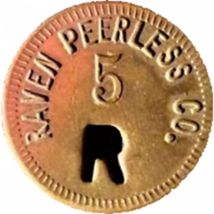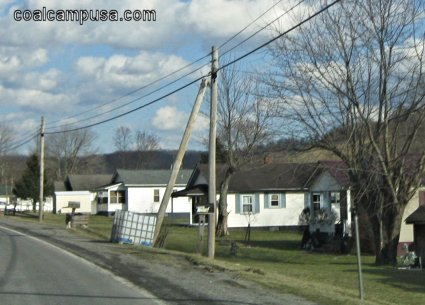Feb. 2016 image by author

Remaining coal company houses along Route 55.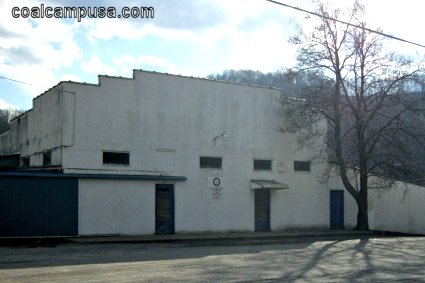Feb. 2016 image by author

Former Raven-Peerless Company store.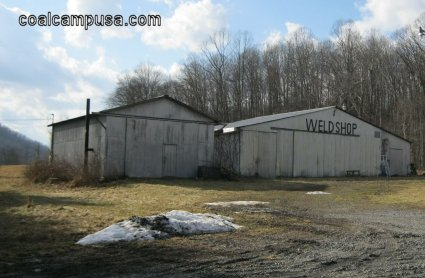Feb. 2016 image by author

These buildings may be left over from the mine.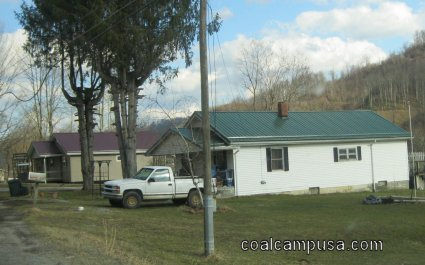Feb. 2016 image by author

SOUTHERN WEST VIRGINIA COALFIELDS

APPALACHIAN COALFIELDS HOME

BECK LEY P O S T -H E R A L D . B EC K L E Y , W . V A ., T H U R S D A Y MORNING, J U L Y 18. 1916 RAVEN PEERLESS CO, INCORPORATED BY BECKLEY PEOPLE S U M M E R S V I L L E , J u l y 17 � T h r e e B e c k l e y p eo p le h a v e o r g a n ized a c o r p o r a t i o n to c a r r y on f u t u r e b u si ne s s o p e r a t i o n s in N i c h olas C o u n t y u n d e r t h e n a m e o f the R a v e n P e e r l e s s C o m p a n y . I n c o r p o r a t o r s of the \$150,000 b us i ne s s , ac o r b i n g to t he S e c r e t a r y of s t ate, a r e E. S. P u s h , jr . , J o h n R H o r n b r o o k a n d M a r y B. H o r n b r o o k , all of Beckley. H o r n b r o o k said t h e c o m p a n y , w h i c h a t t h e p r e s e n t t i m e " o w n s n o t h i n g " h a s b e e n o r g a n i z e d f o r f u t u r e t r a n s a c t i o n s in r e a l e s t a t e a n d b u i l d i n g a n d poss ibl y m e r c h a n d i s i n g in t h e N i c h o l a s c o a l fields n e a r H oo k er s v i ll e. F. S. P u g h , sr., p r e s i d e n t o f Ralei gh S m o k e l e s s F ue l C o m p an y, sal es a g e n c y for t h e L i l l y b r o o k C o al C o m p a n y , f i r m s r e c e nt l y sold to t h e B l a c k s t o n e C o a l C o m p a n y , a M a r y l a n d c o r p o r a tion, still is a s s o c i at e d in N i c h o l a s C o u n t y w i t h d e v e l o p m e n t of R a v en Coals. Inc. E. S. Pugh, Sr., president of the Raven Peerless company, has announced the purchase of the i Campbell coal mine at McMillions Creek. The transaction was made I Saturday The mine formerly was owned S:-^Uge"e , C;.a mpbe;-l-l;,/ Gauley I,Bllage- Pr�duction of the Peer less Seam began m Ma* 1939- and the mine now produces approximately 200 tons per shift.